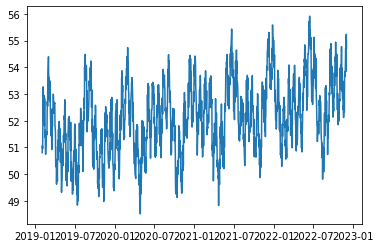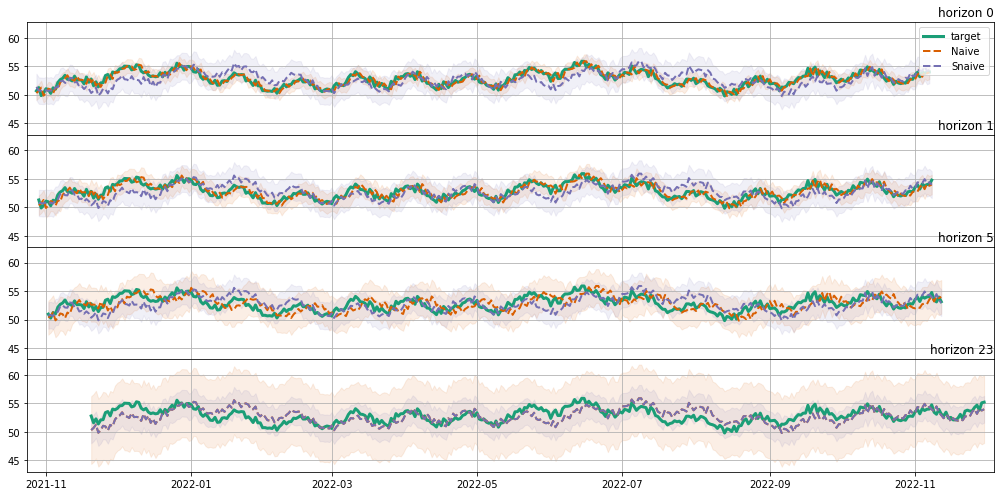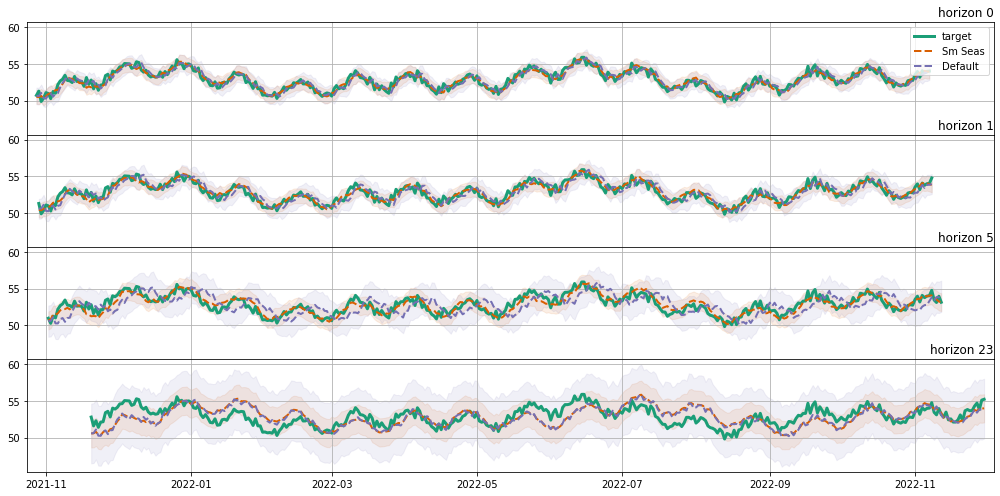In :
import pandas as pd
import numpy as np
import random

import statsmodels.api as sm

from datetime import datetime, timedelta

import matplotlib.pyplot as plt
import matplotlib

from pandas.plotting import register_matplotlib_converters
register_matplotlib_converters()

from ts_eval.viz import metrics as mtx
from ts_eval.viz import time_slices
from ts_eval.viz.api import TSMetrics
from ts_eval.viz.data_containers import xr_2d_factory
from ts_eval.models.naive import snaive_pi
from ts_eval.forecast_strategy.naive import NaiveForecastStrategy, SNaiveForecastStrategy
from ts_eval.forecast_strategy.sm import SMForecastStrategy
from ts_eval.utils import create_sliding_dataset

from ts_eval.utils import seasonality, trend

np.random.seed(0)
matplotlib.rcParams['figure.figsize'] = (14, 4)


# Data¶

Let's create some fake data first. It should contain trend, double seasonality and some noise.

In :
level = 50
n = 1400
period1 = 24
period2 = 24*7
H = 24
train_test_split_idx = 1000

ts = (level +
trend(n, steepness=0.9) +
seasonality(n, period1) +
seasonality(n, period2))

sd = datetime(2019,2,1,0,0,0)

ts = pd.DataFrame(ts, index=pd.date_range(start=sd, end=sd + timedelta(days=ts.shape-1), freq='1d'))

ts_train, ts_test = ts.iloc[:train_test_split_idx], ts.iloc[train_test_split_idx:]

ts_train.shape, ts_test.shape

Out:
((1000, 1), (400, 1))

Here's what the data looks like. A slight upward trend can be observed.

In :
plt.plot(ts, linestyle='-');# Baseline¶

As we know, the data contains double seasonality. Still, let's compute both naive and snaive forecasts using a helper function:

In :
naive = NaiveForecastStrategy(ts, train_test_split_index=train_test_split_idx, freq=1).forecast(h=H).xarray()
snaive = SNaiveForecastStrategy(ts, train_test_split_index=train_test_split_idx, freq=24).forecast(h=H).xarray()

naive.mean_.shape, snaive.mean_.shape

Out:
((400, 24), (400, 24))
In :
target = xr_2d_factory(create_sliding_dataset(ts_test, h=H).squeeze(),
start_date=ts_test.index,
freq=ts_test.index.freq)

target.mean_.shape

Out:
(400, 24)

Just for the sake of demonstration, let's compare naive and snaive forecasts for out dataset:

In :
(TSMetrics(target, naive, snaive)
.for_horizons(0, 1, 5, 23)
.for_time_slices(time_slices.all, time_slices.weekend)
.with_description()
.with_metrics(mtx.MSE, mtx.MIS)
.with_predictions_plot()
.show())

Out:
Date: Wed, 16 Oct 2019 14:25:02 400 24 4ybed4tVsI FMmfz2B5N0 BCGyVo9s3zMSE MIS 1.81 8.16 0.373 2.63 0.482 3.43 1.44 5.96 1.53 11.9
MSE MIS 1.53 5.25 1.53 5.23 1.53 5.23 1.53 5.24 1.53 5.25
MSE MIS 1.74 8.16 0.419 2.74 0.487 3.43 1.37 5.94 1.49 11.9
MSE MIS 1.49 5.52 1.48 5.50 1.48 5.50 1.49 5.52 1.49 5.52
 – ranked better(statistically) – similar rank(statistically) * – not different fromreference (statistically)

Of course, snaive forecast is much better. Let's set it as a baseline.

# SARIMAX Basic Model¶

Now we can use something more advanced. Let's assume we already did some kind of model properties selection (either AIC/BIC based, or CV/validation based).

So our model is SARIMAX(2, 1, 1)x(1, 0, 1, 24).

In :
SARIMAX_DEF = {"order": (2, 1, 1), "seasonal_order": (1,0,1,24)}
model = sm.tsa.SARIMAX(ts_train, **SARIMAX_DEF)
model_fit = model.fit()

/Users/vshulyak/.local/share/virtualenvs/ts-eval-VPVj6ULy/lib/python3.6/site-packages/statsmodels/tsa/statespace/sarimax.py:949: UserWarning: Non-stationary starting autoregressive parameters found. Using zeros as starting parameters.
warn('Non-stationary starting autoregressive parameters'
/Users/vshulyak/.local/share/virtualenvs/ts-eval-VPVj6ULy/lib/python3.6/site-packages/statsmodels/tsa/statespace/sarimax.py:961: UserWarning: Non-invertible starting MA parameters found. Using zeros as starting parameters.
warn('Non-invertible starting MA parameters found.'

In :
model_fit.summary()

Out:
Dep. Variable: No. Observations: 0 1000 SARIMAX(2, 1, 1)x(1, 0, 1, 24) -733.445 Wed, 16 Oct 2019 1478.889 14:25:27 1508.330 02-01-2019 1490.079 - 10-27-2021 opg
coef std err z P>|z| [0.025 0.975] -0.1137 0.037 -3.090 0.002 -0.186 -0.042 -0.0737 0.027 -2.779 0.005 -0.126 -0.022 -0.5637 0.039 -14.341 0.000 -0.641 -0.487 0.9996 0.001 813.454 0.000 0.997 1.002 -0.9723 0.038 -25.615 0.000 -1.047 -0.898 0.2380 0.014 17.078 0.000 0.211 0.265
 Ljung-Box (Q): Jarque-Bera (JB): 75.34 3.15 0 0.21 0.84 0.07 0.12 2.76

Warnings:
 Covariance matrix calculated using the outer product of gradients (complex-step).

Now we can apply sliding window strategy and update the model using kalman filter smoothing:

In :
sm_seas = SMForecastStrategy(model_fit, SARIMAX_DEF).forecast(
ts_test, test_exog=None, h=H
).xarray()

sm_seas.mean_.shape

Out:
(400, 24)
In :
SARIMAX_DEF = {"order": (2, 1, 1)}
model = sm.tsa.SARIMAX(ts_train, **SARIMAX_DEF)
model_fit = model.fit()

default = SMForecastStrategy(model_fit, SARIMAX_DEF).forecast(
ts_test, test_exog=None, h=H
).xarray()

default.mean_.shape

Out:
(400, 24)

Now we can compare the model with snaive baseline:

In :
(
TSMetrics(target, sm_seas, default)
.use_reference(snaive)
.for_horizons(0, 1, 5, 23)
.for_time_slices(time_slices.all, time_slices.weekend)
.with_description()
.with_prediction_rankings(mtx.FVrMSE, mtx.FVrMIS)
.with_predictions_plot()
.show()
)

Out:
Date: Wed, 16 Oct 2019 14:25:36 400 24 Snaive 4ybed4tVsI qeIGs24vG6 cSOa6u9gKF BCGyVo9s3zSm Seas Default 0.514 -0.144 0.856 0.789 0.847 0.706 0.781 0.0457* -0.0149* 0.0290*
Sm Seas Default 0.322 -0.137 0.600 0.530 0.581 0.445 0.492 0.0950 -0.0168 -0.555
Sm Seas Default 0.515 -0.130 0.848 0.761 0.852 0.689 0.776 0.0770* -0.0207* 0.0227*
Sm Seas Default 0.356 -0.0796 0.617 0.536 0.589 0.460 0.502 0.123 0.0504 -0.479
 – ranked better(statistically) – similar rank(statistically) * – not different fromreference (statistically)

Clearly, overall performance for SARIMAX is better, however on point 23 the performance of naive forecast is indistinguishable from sarimax. Great, so the model can be made better!